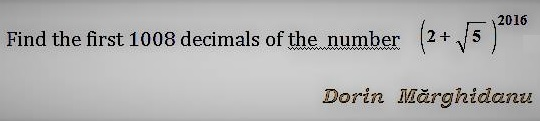# First 1008 Digits

### Problem### Solution 1

$0\lt\sqrt{5}-2\lt\displaystyle\frac{1}{4}\,$ so that $\displaystyle\left(\sqrt{5}-2\right)^2\lt\frac{1}{16}\lt\frac{1}{10}.\,$ It follows that $\left(2-\sqrt{5}\right)^{2016}\lt 10^{-1008}.\,$ But $(2+\sqrt{5})^{2016}+(2-\sqrt{5})^{2016}=n\in\mathbb{N},\,$ implying $(2+\sqrt{5})^{2016}=n-(2-\sqrt{5})^{2016}\gt n-10^{-1008}.$

Hence, each of the first 1008 digits is equal to $9.$

### Solution 2### Solution 3

Setting $\alpha =2+\sqrt{5}$, it easy to see that $\alpha =\varphi ^{3}$, where $\varphi$ is the golden ratio, so we have that $\alpha ^{2016}=\varphi ^{6048}.$ A very well known property of the golden ratio is that $\varphi ^{2k}+\frac{1}{\varphi ^{2k}}$ is an integer for every $k\geq 0.$ So we have that $\alpha ^{2016}=n-\frac{1}{\varphi ^{6048}}$ for some positive integer $n$. Now a direct computation shows that $\varphi ^{6}=(\frac{1+\sqrt{5%}}{2})^{6}>10$, so that $\varphi ^{6048}>10^{1008},$ that is $\frac{1}{\varphi ^{6048}}<\frac{1}{10^{1008}}.$

This inequality shows that the first $%1008$ decimals of $\alpha ^{2016}$ are all $9$.

### Acknowledgment

The problem above has been kindly posted at the CutTheKnotMath facebook page by Dorin Marghidanu. Solution 1 is by Leo Giugiuc; Solution 2 is by Dorin Marghidanu; Solution 3 is by Giulio Francot.

### Remark

The problem is easily modifiable; the fact that it relates to the Golden ratio is utterly irrelevant. For example, a similar question could have been asked about, say $2+\sqrt{3}\,$ or $1+\sqrt{2}.\,$

In addition, the problem allows for a refinement. From Solution 1, $\displaystyle\left(\sqrt{5}-2\right)^2\lt\frac{1}{16}\lt\frac{1}{10}\,$ and then $\displaystyle\left(\sqrt{5}-2\right)^6\lt\frac{1}{10^3}.\,$ However, as a matter of fact, already $\displaystyle\left(\sqrt{5}-2\right)^5\lt\frac{1}{10^3}.\,$ It follows that

$\displaystyle\left(\sqrt{5}-2\right)^{2016}\lt\left(\sqrt{5}-2\right)^{2015}\lt\frac{1}{10^{1209}}\,$

implying that $\displaystyle\left(2+\sqrt{5}\right)^{2016}\,$ has at least $1209\,$ first digits all equal $9!\,$

Furthermore, according to wolframalpha, $(\sqrt{5}-2)^{2016}\lt 1.103477\ldots\times 10^{-1264},\,$ such that $\displaystyle\left(2+\sqrt{5}\right)^{2016}\,$ has in fact $1263\,$ decimal digits $9.\,$ It would be interesting to discover this approximation by some kind of factoring or splitting $2016\,$ or a close number, as we just did.